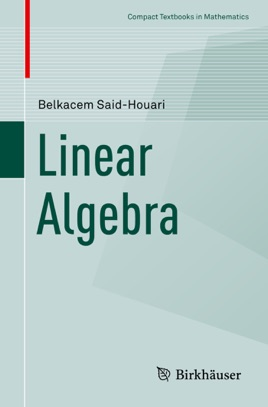• \$44.99

## Publisher Description

This self-contained, clearly written textbook on linear algebra is easily accessible for students. It begins with the simple linear equation and generalizes several notions from this equation for the system of linear equations and introduces the main ideas using matrices. It then offers a detailed chapter on determinants and introduces the main ideas with detailed proofs. The third chapter introduces the Euclidean spaces using very simple geometric ideas and discusses various major inequalities and identities. These ideas offer a solid basis for understanding general Hilbert spaces in functional analysis. The following two chapters address general vector spaces, including some rigorous proofs to all the main results, and linear transformation: areas that are ignored or are poorly explained in many textbooks. Chapter 6 introduces the idea of matrices using linear transformation, which is easier to understand than the usual theory of matrices approach. The final two chapters are more advanced, introducing the necessary concepts of eigenvalues and eigenvectors, as well as the theory of symmetric and orthogonal matrices. Each idea presented is followed by examples.  The book includes a set of exercises at the end of each chapter, which have been carefully chosen to illustrate the main ideas. Some of them were taken (with some modifications) from recently published papers, and appear in a textbook for the first time. Detailed solutions are provided for every exercise, and these refer to the main theorems in the text when necessary, so students can see the tools used in the solution.